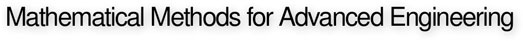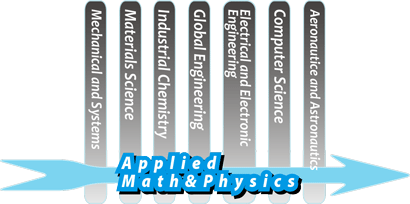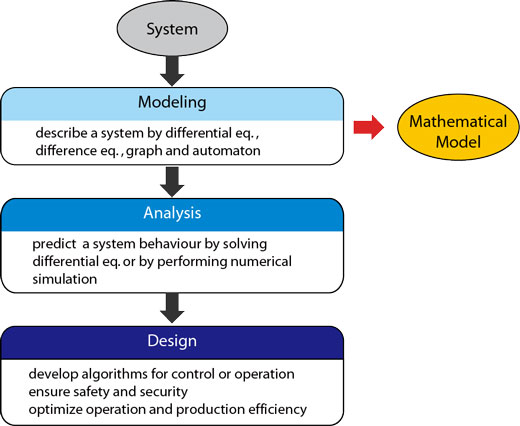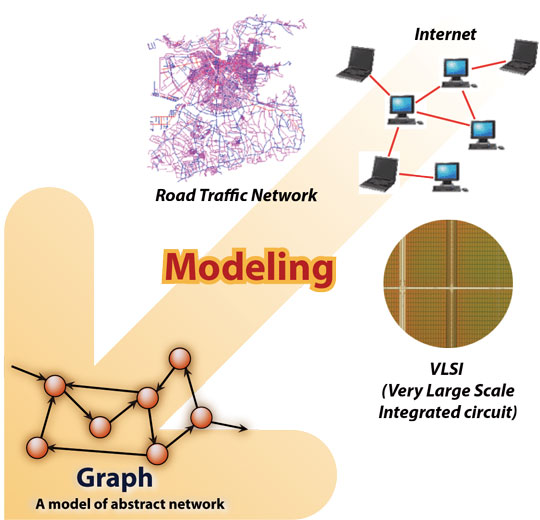Home / What is Applied Mathematics and Physics?

# What is Applied Mathematics and Physics?## Goals of Applied Mathematics and Physics

Systems around us have become more and more complicated and enormous in scale these days. These systems are a mix of technologies from various disciplines. What is vital for the safe and efficient operation and control of these systems is interdisciplinary mathematical thinking to find solutions for problems focusing on the entire mathematical structure of these systems.In the Applied Mathematics and Physics Course, we conduct education and research based on mathematics and physics aided by computers with a goal of seeking the methods to resolve engineering problems in various fields including information science. To this end, we are solving the mechanisms of creation, conversion, transfer, systematization, optimization, and control of information in dynamically changing complicated systems.

## Features of AMP

The processes to resolve engineering problems are composed of "Modeling," "Analysis" and "Design."Applied Mathematics and Physics is a study to theoretically seek the methodologies of these three processes. Its distinctive feature lies in providing universal methods and algorithms for resolving problems based on abstract mathematical models focusing on common structures of these processes, rather than in using methods unique to each actual engineering problem. In other words, if an excellent solution for a question expressed by an abstract mathematical model is discovered, the solution will be applicable to any problem with the same mathematical structure.

### Example: networks

• Actual networks: road networks, railway networks, computer networks, electronic networks, ....
• Common structure:
• Graphs, collections of nodes and edges (points and lines).
• Nodes (points): intersections of roads, railway stations, PCs and routers, semiconductor chips, ....
• Edges (lines): roads, railways, communication lines, electric wires, ....
• Traffic in road or railway networks, data packets in computer networks, currents in electronic networks, ... flow via the nodes and through the edges.

If it is possible to contrive analytic and design methods for abstract graphs, the results can be applied to diversified actual networking. Such a discipline concerning graphs is called "Graph Theory," and is handled as one of the classes in the Applied Mathematics and Physics Course Curriculum.This example shows modeling of a system (network) using a graph. In many cases, target systems are described using mathematical formulas such as inequalities and equations (differential equations/recurrence formulas/algebraic equations) depending on the situation. In any case, seeking universal methods based on mathematical models is a common feature of every research topic in Applied Mathematics and Physics.

## AMP with Computer

In the Applied Mathematics and Physics course, we conduct education and research making full use of computers. In other words, we conduct modeling and analysis of systems through computer-aided data processing and simulation, as well as the design of algorithms and controllers for computers. In this way, computers have become an important tool to realize the methodologies of Applied Mathematics and Physics. The Applied Mathematics and Physics Course also provides a quality curriculum for Computer Practice and Information Science as a course in the School of Informatics and Mathematical Science.

## AMP Symposium

Please see this page for further information.